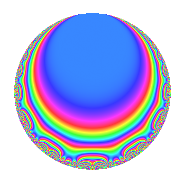# Properties

 Label 72.2.fLevel 72 Weight 2 Character orbit f Rep. character $$\chi_{72}(35,\cdot)$$ Character field $$\Q$$ Dimension 4 Newforms 1 Sturm bound 24 Trace bound 0

# Related objects

## Defining parameters

 Level: $$N$$ = $$72 = 2^{3} \cdot 3^{2}$$ Weight: $$k$$ = $$2$$ Character orbit: $$[\chi]$$ = 72.f (of order $$2$$ and degree $$1$$) Character conductor: $$\operatorname{cond}(\chi)$$ = $$24$$ Character field: $$\Q$$ Newforms: $$1$$ Sturm bound: $$24$$ Trace bound: $$0$$

## Dimensions

The following table gives the dimensions of various subspaces of $$M_{2}(72, [\chi])$$.

Total New Old
Modular forms 16 4 12
Cusp forms 8 4 4
Eisenstein series 8 0 8

## Trace form

 $$4q$$ $$\mathstrut +\mathstrut 4q^{4}$$ $$\mathstrut +\mathstrut O(q^{10})$$ $$4q$$ $$\mathstrut +\mathstrut 4q^{4}$$ $$\mathstrut -\mathstrut 12q^{10}$$ $$\mathstrut -\mathstrut 8q^{16}$$ $$\mathstrut -\mathstrut 16q^{19}$$ $$\mathstrut +\mathstrut 8q^{22}$$ $$\mathstrut +\mathstrut 4q^{25}$$ $$\mathstrut +\mathstrut 24q^{28}$$ $$\mathstrut -\mathstrut 4q^{34}$$ $$\mathstrut +\mathstrut 32q^{43}$$ $$\mathstrut +\mathstrut 24q^{46}$$ $$\mathstrut -\mathstrut 20q^{49}$$ $$\mathstrut -\mathstrut 24q^{52}$$ $$\mathstrut +\mathstrut 12q^{58}$$ $$\mathstrut -\mathstrut 32q^{64}$$ $$\mathstrut -\mathstrut 16q^{67}$$ $$\mathstrut -\mathstrut 24q^{70}$$ $$\mathstrut -\mathstrut 16q^{73}$$ $$\mathstrut -\mathstrut 16q^{76}$$ $$\mathstrut -\mathstrut 4q^{82}$$ $$\mathstrut +\mathstrut 32q^{88}$$ $$\mathstrut +\mathstrut 48q^{91}$$ $$\mathstrut -\mathstrut 24q^{94}$$ $$\mathstrut +\mathstrut 32q^{97}$$ $$\mathstrut +\mathstrut O(q^{100})$$

## Decomposition of $$S_{2}^{\mathrm{new}}(72, [\chi])$$ into irreducible Hecke orbits

Label Dim. $$A$$ Field CM Traces $q$-expansion
$$a_2$$ $$a_3$$ $$a_5$$ $$a_7$$
72.2.f.a $$4$$ $$0.575$$ $$\Q(\sqrt{-2}, \sqrt{-3})$$ None $$0$$ $$0$$ $$0$$ $$0$$ $$q+\beta _{1}q^{2}+(1+\beta _{3})q^{4}+(-2\beta _{1}+\beta _{2}+\cdots)q^{5}+\cdots$$

## Decomposition of $$S_{2}^{\mathrm{old}}(72, [\chi])$$ into lower level spaces

$$S_{2}^{\mathrm{old}}(72, [\chi]) \cong$$ $$S_{2}^{\mathrm{new}}(24, [\chi])$$$$^{\oplus 2}$$